## Appendix E. Available plot marks

Plot marks can be added to almost all linear (cartesian) graph types. They are used to emphasise the actual data points in the graph. The plot mark property is available as the instance variable "`\$mark`" for the plot types that support plot marks.

## E.1. Built in basic plot marks

To embellishment a line plot with one of the built-in basic plot marks, say a a red diamond mark, at each data point the following line has to be added to the graph script

 ```1 2 3 4 ``` ```mark->SetType(MARK_DIAMOND); \$lineplot->mark->SetFillColor('red'); ?>```

The rest of the basic plot marks are handled analogues.

Table E.1. Built in line based plot marks

Displayed plot markSymbolic name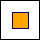`MARK_SQUARE``MARK_UTRIANGLE`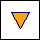`MARK_DTRIANGLE``MARK_DIAMOND``MARK_CIRCLE`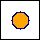`MARK_FILLEDCIRCLE`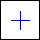`MARK_CROSS`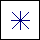`MARK_STAR``MARK_X``MARK_LEFTTRIANGLE``MARK_RIGHTTRIANGLE``MARK_FLASH`